# General Report on Tunny

21 Page 38

o =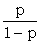. The odds of an event E given an hypothesis H are written as O(E|H). Sometimes odds are expressed as a ratio such as '3:2' or '3 to 2'. This means o=3/2. The following phrases are equivalent.
'a:b', 'a:b on', 'o = a/b', 'a to b', 'b to a against' etc.

(c) Special values of p.

 'Certainty''Impossibility''Evens' p=1 or o=∞p=0 or o=0p=½ or o=1.

(d) Relationship of Events.

Two events are 'mutually exclusive' if they cannot both happen. Two events are 'independent' if a knowledge that one is true does not affect the probability of the other one. A number of events is 'exhaustive' if it is certain that one or other of them will happen.

(e) The laws of probability.

 (i) the law of addition of probability P(E1∨E2|H) = P(E1|H) + P(E2|H) if E1 and E2 are mutually exclusive. (ii) the law of multiplication of probabilities P(E1E2|H) = P(E1|H) P(E2|E1H). In particular, if E1 and E2 are independent P(E1E2|H) = P(E1|H) P(E2|H)

(f) Some theorems.

 (i) P(E1E2 ... En|H) =  P(E1|H) P(E2|E1H) P(E3|E1E2H) ... P(En|(E1 ... En-1H). (ii) P(E1∨E2∨ ... ∨En|H)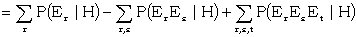- ... etc.,

and in particular if E1, E2, ..., En are all mutually exclusive, the right hand side can be replaced by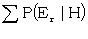. If E1∨ ... ∨Er is exhaustive the left hand side is 1. Therefore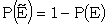.

 (iii) Bayes' theorem. For various hypotheses Hi (i = 1, 2...)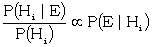The proof of this is simple. For by the law of multiplication of probabilities.